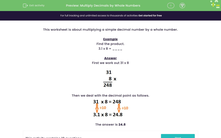# Multiply Decimals by Whole Numbers

In this worksheet, students multiply a simple decimal number by a whole number.Key stage:  KS 2

Curriculum topic:   Number: Multiplication and Division

Curriculum subtopic:   Multiply/Divide Using Decimals

Difficulty level:#### Worksheet Overview

This worksheet is about multiplying a simple decimal number by a whole number.

Example

Find the product.

3.1 x 8 = ____

First we work out 31 x 8Then we deal with the decimal point as follows.